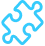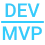Solved

# Tax Option for discounts

• 5 replies
• 190 views

• Freshman I
• 2 replies

When creating any kind of discount code we need option to determine if it should be pre tax or post tax Every discount now is calculated before tax.

This exists in every other product we compete against.

icon

Best answer by Irina 24 November 2020, 15:05

View original

### 5 replies

Userlevel 7+7

It looks like we have this as an idea in our Feedback system, please check: cash discounts - allow calculation on base amount.

Userlevel 7+7

@barryk77, please advise, maybe this is what you've been searching for?

Defining How Cash Discounts Are Applied

A cash discount can be applied to a document only within the cash discount period. The end date of the cash discount period is specified in the Cash Discount Date box in the invoice (or the similar document). This date is defined by the system according to the specified credit terms. For details, see Setup of Credit Terms.

In the Cash Discount box on the Taxes form, you can specify the method of calculating the taxable amount if a cash discount is applied to the document. You can select one of the following options:

• Reduces Taxable Amount: The system deducts the discount amount from the document amount before tax calculation. Thus, the tax amount is calculated based on the discounted taxable amount. For example, assume that the price of the one purchased item is \$100, the tax rate is 10%, and the cash discount is 20%. In this case, the taxable amount is decreased by the discount amount and is equal to \$100 (price) - \$20 (discount) = \$80. Thus, the tax amount is \$8. The document amount is equal to the product price plus the discounted tax amount: \$100 (price) + \$8 (tax amount) = \$108.
• Does Not Affect Taxable Amount (selected by default): The discount amount doesn’t affect the taxable amount. That is, the base for tax calculation is the same as the base for discount calculation. As in the previous example, consider a price of \$100, a discount of 20%, and a tax of 10%. Because the taxable amount is not affected by the discount, it is \$100. The tax amount is \$10. Thus, the document amount is \$110.
• Reduces Taxable Amount on Early Payment (this option is available for the taxes of the VAT type only). The system recalculates the taxable amount in the document if the full payment has been received within the cash discount period. The documents that have been fully paid within the cash discount period are listed on the Generate VAT Credit Memos (AR504500) form. On this form, you can generate credit memos that will be automatically applied to the corresponding documents upon release. For details, see VAT Calculated for Documents with Cash Discounts.

Irina:

How is this the same?

I am not talking about cash discounts. I am talking about discounts here -

ScreenId=AR2095PL

Thank you

Userlevel 7+7

Irina:

How is this the same?

I am not talking about cash discounts. I am talking about discounts here -

ScreenId=AR2095PL

Thank you

Understood. Currently, there is no option to set this up. This option is only available for cash discounts as described above.

I suggest creating an idea in https://community.acumatica.com/ideas

Userlevel 5+1

Yes, if this feature is implemented in Acumatica then that would be very helpful.